# 2.2 Graphs of linear functions  (Page 10/15)

 Page 10 / 15

$\begin{array}{c}3y+x=12\\ -y=8x+1\end{array}$

neither parallel or perpendicular

$\begin{array}{c}3y+4x=12\\ -6y=8x+1\end{array}$

$\begin{array}{c}6x-9y=10\\ 3x+2y=1\end{array}$

perpendicular

$\begin{array}{c}y=\frac{2}{3}x+1\\ 3x+2y=1\end{array}$

$\begin{array}{c}y=\frac{3}{4}x+1\\ -3x+4y=1\end{array}$

parallel

For the following exercises, find the x - and y- intercepts of each equation

$f\left(x\right)=-x+2$

$g\left(x\right)=2x+4$

;

$h\left(x\right)=3x-5$

$k\left(x\right)=-5x+1$

;

$-2x+5y=20$

$7x+2y=56$

;

For the following exercises, use the descriptions of each pair of lines given below to find the slopes of Line 1 and Line 2. Is each pair of lines parallel, perpendicular, or neither?

• Line 1: Passes through $\left(0,6\right)$ and $\left(3,-24\right)$
• Line 2: Passes through $\left(-1,19\right)$ and $\left(8,-71\right)$
• Line 1: Passes through $\left(-8,-55\right)$ and $\left(10,\text{\hspace{0.17em}}89\right)$
• Line 2: Passes through $\left(9,-44\right)$ and $\left(4,-14\right)$

• Line 1: Passes through $\left(2,3\right)$ and $\left(4,-1\right)$
• Line 2: Passes through $\left(6,3\right)$ and $\left(8,5\right)$
• Line 1: Passes through $\left(1,7\right)$ and $\left(5,5\right)$
• Line 2: Passes through $\left(-1,-3\right)$ and $\left(1,1\right)$

• Line 1: Passes through $\left(0,5\right)$ and $\left(3,3\right)$
• Line 2: Passes through $\left(1,-5\right)$ and $\left(3,-2\right)$
• Line 1: Passes through $\left(2,5\right)$ and $\left(5,-1\right)$
• Line 2: Passes through $\left(-3,7\right)$ and $\left(3,-5\right)$

Write an equation for a line parallel to $f\left(x\right)=-5x-3$ and passing through the point

Write an equation for a line parallel to $g\left(x\right)=3x-1$ and passing through the point $\left(4,9\right).$

$g\left(x\right)=3x-3$

Write an equation for a line perpendicular to $h\left(t\right)=-2t+4$ and passing through the point

Write an equation for a line perpendicular to $p\left(t\right)=3t+4$ and passing through the point $\left(3,1\right).$

$p\left(t\right)=-\frac{1}{3}t+2$

Find the point at which the line $f\left(x\right)=-2x-1$ intersects the line $g\left(x\right)=-x.$

Find the point at which the line $f\left(x\right)=2x+5$ intersects the line $g\left(x\right)=-3x-5.$

$\left(-2,1\right)$

Use algebra to find the point at which the line intersects the line $h\left(x\right)=\frac{9}{4}x\text{\hspace{0.17em}}\text{\hspace{0.17em}}+\text{\hspace{0.17em}}\text{\hspace{0.17em}}\frac{73}{10}.$

Use algebra to find the point at which the line $f\left(x\right)=\frac{7}{4}x\text{\hspace{0.17em}}\text{\hspace{0.17em}}+\text{\hspace{0.17em}}\text{\hspace{0.17em}}\frac{457}{60}$ intersects the line $g\left(x\right)=\frac{4}{3}x\text{\hspace{0.17em}}\text{\hspace{0.17em}}+\text{\hspace{0.17em}}\text{\hspace{0.17em}}\frac{31}{5}.$

$\left(-\frac{17}{5},\frac{5}{3}\right)$

## Graphical

For the following exercises, the given linear equation with its graph in [link] .

$f\left(x\right)=-x-1$

$f\left(x\right)=-2x-1$

F

$f\left(x\right)=-\frac{1}{2}x-1$

$f\left(x\right)=2$

C

$f\left(x\right)=2+x$

$f\left(x\right)=3x+2$

A

For the following exercises, sketch a line with the given features.

An x -intercept of and y -intercept of

An x -intercept of and y -intercept of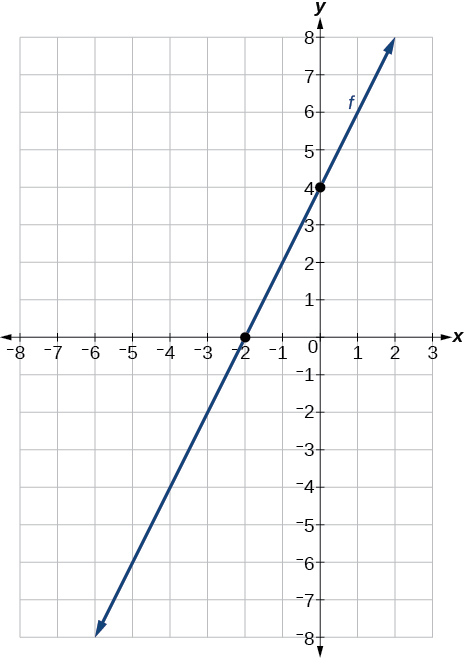A y -intercept of and slope $-\frac{3}{2}$

A y -intercept of and slope $\frac{2}{5}$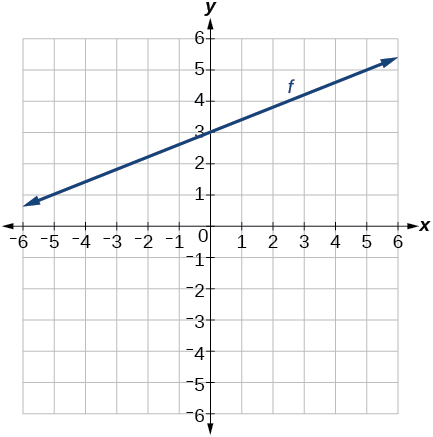Passing through the points and

Passing through the points and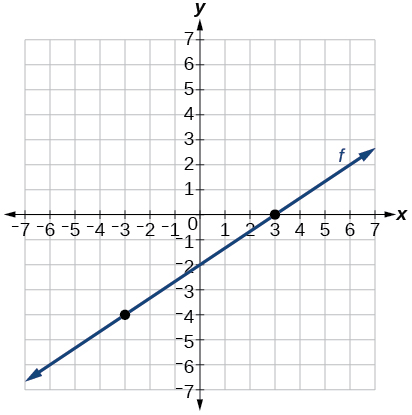For the following exercises, sketch the graph of each equation.

$f\left(x\right)=-2x-1$

$g\left(x\right)=-3x+2$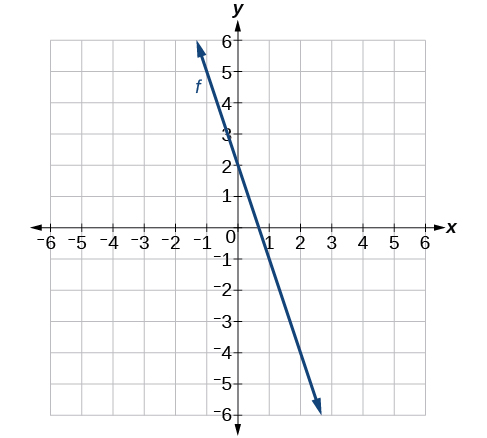$h\left(x\right)=\frac{1}{3}x+2$

$k\left(x\right)=\frac{2}{3}x-3$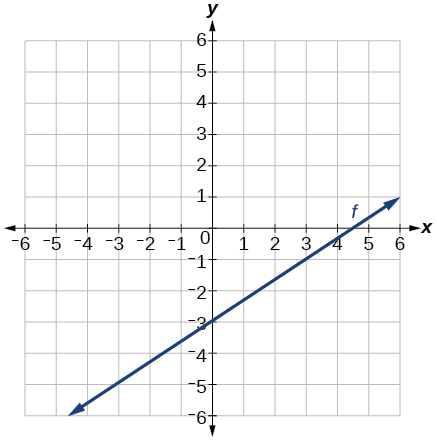$f\left(t\right)=3+2t$

$p\left(t\right)=-2\text{\hspace{0.17em}}+\text{\hspace{0.17em}}3t$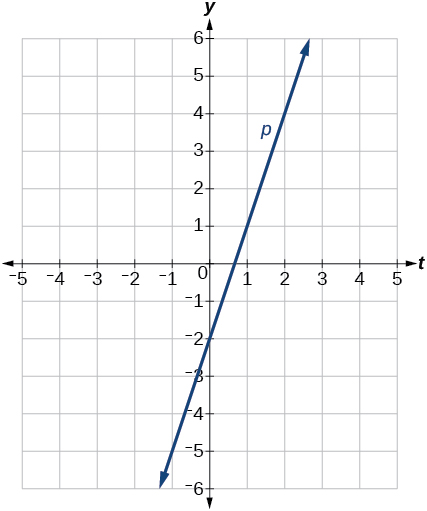$x=3$

$x=-2$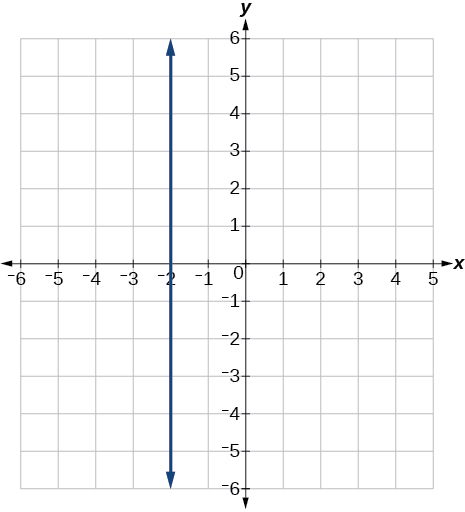$r\left(x\right)=4$

$q\left(x\right)=3$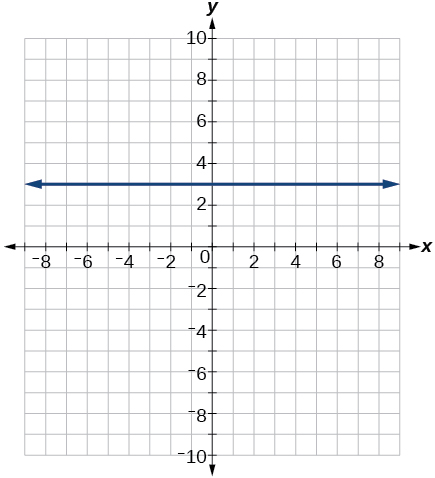$4x=-9y+36$

$\frac{x}{3}-\frac{y}{4}=1$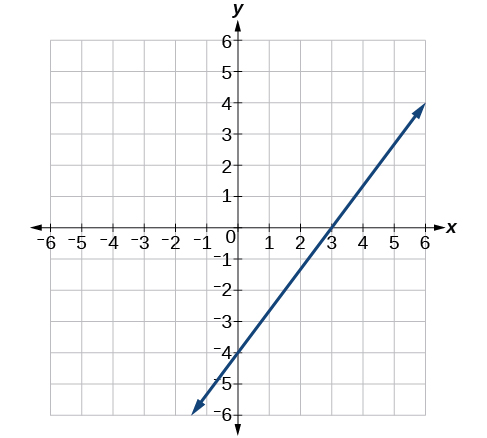$3x-5y=15$

$3x=15$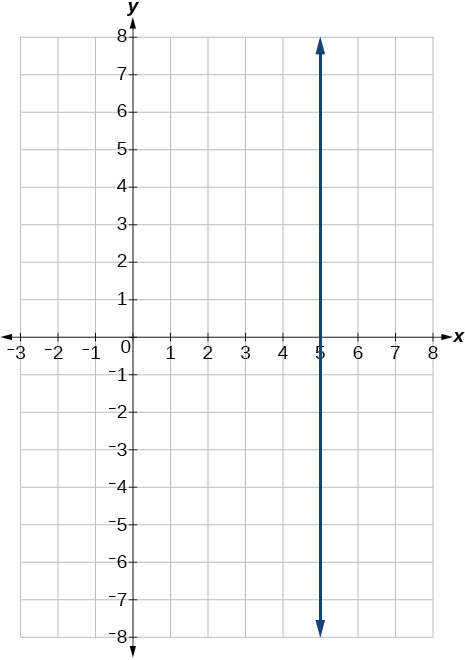$3y=12$

If $g\left(x\right)$ is the transformation of $f\left(x\right)=x$ after a vertical compression by $\frac{3}{4},$ a shift right by 2, and a shift down by 4

1. Write an equation for $g\left(x\right).$
2. What is the slope of this line?
3. Find the y- intercept of this line.

• $g\left(x\right)=0.75x-5.5\text{}$
• 0.75
• $\left(0,-5.5\right)$

If $g\left(x\right)$ is the transformation of $f\left(x\right)=x$ after a vertical compression by $\frac{1}{3},$ a shift left by 1, and a shift up by 3

1. Write an equation for $g\left(x\right).$
2. What is the slope of this line?
3. Find the y- intercept of this line.

For the following exercises,, write the equation of the line shown in the graph.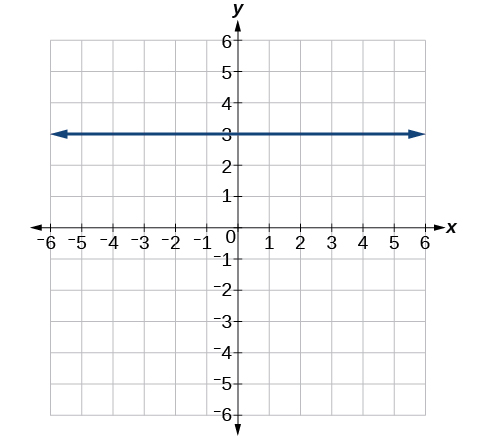$y=\text{3}$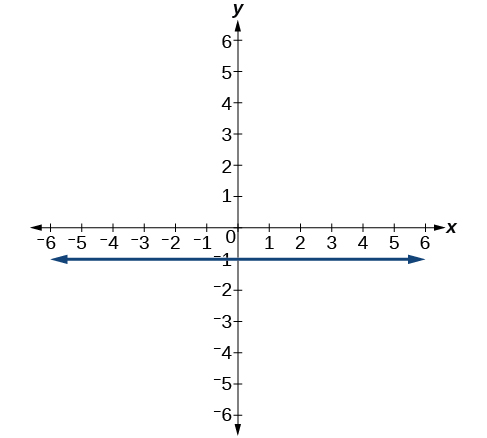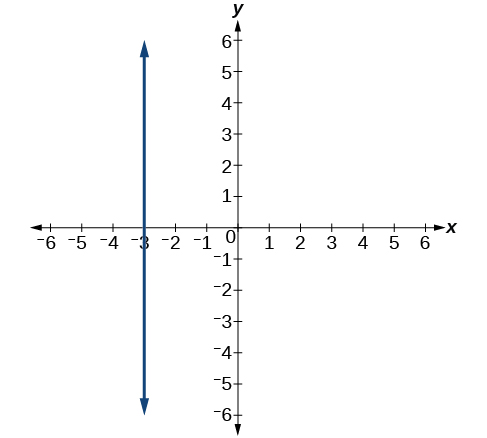$x=-3$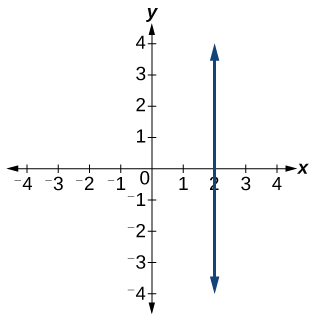For the following exercises, find the point of intersection of each pair of lines if it exists. If it does not exist, indicate that there is no point of intersection.

$\begin{array}{c}y=\frac{3}{4}x+1\\ -3x+4y=12\end{array}$

no point of intersection

$\begin{array}{c}2x-3y=12\\ 5y+x=30\end{array}$

$\begin{array}{c}2x=y-3\\ y+4x=15\end{array}$

$\begin{array}{c}x-2y+2=3\\ x-y=3\end{array}$

$\begin{array}{c}5x+3y=-65\\ x-y=-5\end{array}$

## Extensions

Find the equation of the line parallel to the line $g\left(x\right)=-0.\text{01}x\text{\hspace{0.17em}}\text{+}\text{\hspace{0.17em}}\text{2}\text{.01}$ through the point

Find the equation of the line perpendicular to the line $g\left(x\right)=-0.\text{01}x\text{+2}\text{.01}$ through the point

$y=100x-98$

For the following exercises, use the functions

Find the point of intersection of the lines $f$ and $g.$

Where is $f\left(x\right)$ greater than $g\left(x\right)?$ Where is $g\left(x\right)$ greater than $f\left(x\right)?$

$x<\frac{1999}{201}x>\frac{1999}{201}$

## Real-world applications

A car rental company offers two plans for renting a car.

• Plan A: $30 per day and$0.18 per mile
• Plan B: $50 per day with free unlimited mileage How many miles would you need to drive for plan B to save you money? A cell phone company offers two plans for minutes. • Plan A:$20 per month and $1 for every one hundred texts. • Plan B:$50 per month with free unlimited texts.

How many texts would you need to send per month for plan B to save you money?

Less than 3000 texts

A cell phone company offers two plans for minutes.

• Plan A: $15 per month and$2 for every 300 texts.
• Plan B: $25 per month and$0.50 for every 100 texts.

How many texts would you need to send per month for plan B to save you money?

a colony of bacteria is growing exponentially doubling in size every 100 minutes. how much minutes will it take for the colony of bacteria to triple in size
what is the importance knowing the graph of circular functions?
can get some help basic precalculus
What do you need help with?
Andrew
how to convert general to standard form with not perfect trinomial
can get some help inverse function
ismail
Rectangle coordinate
how to find for x
it depends on the equation
Robert
whats a domain
The domain of a function is the set of all input on which the function is defined. For example all real numbers are the Domain of any Polynomial function.
Spiro
foci (–7,–17) and (–7,17), the absolute value of the differenceof the distances of any point from the foci is 24.
difference between calculus and pre calculus?
give me an example of a problem so that I can practice answering
x³+y³+z³=42
Robert
dont forget the cube in each variable ;)
Robert
of she solves that, well ... then she has a lot of computational force under her command ....
Walter
what is a function?
I want to learn about the law of exponent
explain this
what is functions?
A mathematical relation such that every input has only one out.
Spiro
yes..it is a relationo of orders pairs of sets one or more input that leads to a exactly one output.
Mubita
Is a rule that assigns to each element X in a set A exactly one element, called F(x), in a set B.
RichieRich
If the plane intersects the cone (either above or below) horizontally, what figure will be created?ByBy Robert MurphyBy Ryan LoweBy David CoreyBy Abishek DevarajBy Michael PittBy Richley CrapoBy Anh DaoBy Brooke DelaneyBy Mackenzie WilcoxBy Jazzycazz Jackson